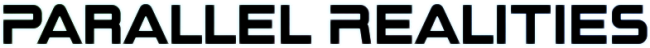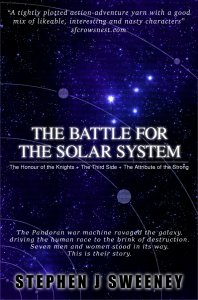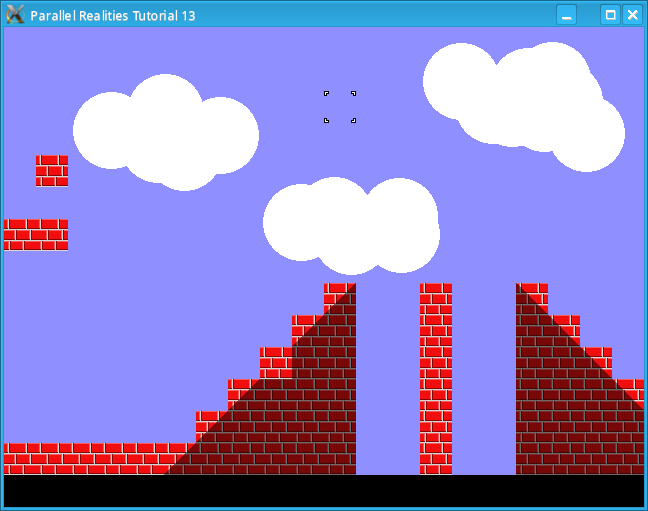Android Games Latest Updates SDL2 Santa game tutorial 🎅 Thu, 23rd November 2023 SDL2 Shooter 3 tutorial Wed, 15th February 2023 The Legend of Edgar 1.36 Sun, 1st January 2023 SDL2 map editor tutorial [UPDATED] Sat, 10th September 2022 TBFTSS: The Pandoran War - Amiga OS4 Port Wed, 7th September 2022 All Updates » Tags • android (3)• battle-for-the-solar-system (10)• blob-wars (10)• brexit (1)• code (6)• edgar (8)• games (40)• lasagne-monsters (1)• making-of (5)• match3 (1)• numberblocksonline (1)• orb (2)• site (1)• tanx (4)• three-guys (3)• three-guys-apocalypse (3)• tutorials (15)• water-closet (4) BooksThe Battle for the Solar System (Complete) The Pandoran war machine ravaged the galaxy, driving the human race to the brink of destruction. Seven men and women stood in its way. This is their story. Intermediate Tutorials Intermediate Game Tutorial #3 - A tile based map editorIntroduction In this tutorial we will create a basic map editor Compile and run tutorial13. The program will read the map data file and display the map on the screen. Use the arrow keys (not the ones on the numeric pad) to scroll the map around. Moving the mouse cursor will move the tile block around. Clicking the left mouse button or pressing space will place a tile at the current mouse position. Clicking the right mouse button will blank the tile at the current map position. Pressing the comma or minus key (not the one on the numeric pad) will select the previous map tile. Pressing the period or plus key (not the one on the numeric pad) will select the next map tile. Pressing S will save the map data and pressing L will load the map data. An in-depth look We have increased the maximum number of tiles to 400 x 300 and also updated the Map structure: ```typedef struct Map { char *filename; int startX, startY; int maxX, maxY; int tile[MAX_MAP_Y][MAX_MAP_X]; SDL_Surface *background; } Map; ``` The filename variable simply stores the path to the map data so that we can load and save it. The background variable is the background image. Currently this is hardcoded but later tutorials will see the filename stored as part of the map data. We also create a structure to handle the tile cursor as follows: ```typedef struct Cursor { int x, y, tileID; } Cursor; ``` The x and y variables are screen coordinates and the tileID is the current ID of the selected tile. We also have a message structure ```typedef struct Message { char text[MAX_MESSAGE_LENGTH]; int counter; } Message; ``` This structure is used to display messages on the screen. The message will be displayed on the screen as long as the counter is greater than 0. The map loading code in map.c has changed to allow dynamic setting of the maximum horizontal and vertical scrolling: ```void loadMap(char *name) { int x, y; FILE *fp; fp = fopen(name, "rb"); /* If we can't open the map then exit */ if (fp == NULL) { printf("Failed to open map %s\n", name); exit(1); } /* Read the data from the file into the map */ map.maxX = map.maxY = 0; for (y=0;y map.maxX) { map.maxX = x; } if (y > map.maxY) { map.maxY = y; } } } } map.maxX++; map.maxY++; /* Set the start coordinates */ map.startX = map.startY = 0; /* Set the maximum scroll position of the map */ map.maxX = MAX_MAP_X * TILE_SIZE; map.maxY = MAX_MAP_Y * TILE_SIZE; /* Set the filename */ map.filename = name; /* Close the file afterwards */ fclose(fp); } ``` Before reading the map data, we set the maxX and maxY values to 0 and then, while reading the map data, we check if the current map value is not the blank tile. If this is true then we check if the current x value is greater than the current maxX value and if it is, then we set it to x. We apply the same logic to the maxY variable. Since this is a map editor however, limiting the scrolling to the bounds of the size of the map would not allow us to expand the map so, we set the size of the map to the maximum possible size. In the next tutorial we will not perform this step. Finally, we set the filename of the map. The save map code is similar in places: ```void saveMap() { int x, y; FILE *fp; fp = fopen(map.filename, "wb"); /* If we can't open the map then exit */ if (fp == NULL) { printf("Failed to open map %s\n", map.filename); exit(1); } /* Write the data from the file into the map */ for (y=0;y= SCREEN_HEIGHT - TILE_SIZE) { cursor.y = SCREEN_HEIGHT - TILE_SIZE * 2; } if (input.left == 1) { map.startX -= TILE_SIZE; if (map.startX < 0) { map.startX = 0; } } else if (input.right == 1) { map.startX += TILE_SIZE; if (map.startX + SCREEN_WIDTH >= map.maxX) { map.startX = map.maxX - SCREEN_WIDTH; } } if (input.up == 1) { map.startY -= TILE_SIZE; if (map.startY < 0) { map.startY = 0; } } else if (input.down == 1) { map.startY += TILE_SIZE; if (map.startY + SCREEN_HEIGHT >= map.maxY) { map.startY = map.maxY - SCREEN_HEIGHT; } } if (input.add == 1) { map.tile[(map.startY + cursor.y) / TILE_SIZE] [(map.startX + cursor.x) / TILE_SIZE] = cursor.tileID; } else if (input.remove == 1) { map.tile[(map.startY + cursor.y) / TILE_SIZE] [(map.startX + cursor.x) / TILE_SIZE] = BLANK_TILE; } if (input.previous == 1) { do { cursor.tileID--; if (cursor.tileID < 0) { cursor.tileID = MAX_TILES - 1; } } while (mapImages[cursor.tileID] == NULL); input.previous = 0; } if (input.next == 1) { do { cursor.tileID++; if (cursor.tileID >= MAX_TILES) { cursor.tileID = 0; } } while (mapImages[cursor.tileID] == NULL); input.next = 0; } if (input.save == 1) { saveMap(); setStatusMessage("Saved OK"); input.save = 0; } if (input.load == 1) { loadMap(map.filename); setStatusMessage("Loaded OK"); input.load = 0; } if (input.left == 1 || input.right == 1 || input.up == 1 || input.down == 1) { SDL_Delay(30); } } ``` First, we prevent the cursor from being able to move into the bottom row of the screen, since we will use that to display messages. We then handle the left, right, up and down arrow key presses as per the previous tutorial. When the add input is 1, we place a tile at the current screen and mouse cursor position. We do this by taking the startX value and adding the cursor.x value and then dividing this value by TILE_SIZE to give us the nearest tile. We do the same for the vertical coordinate. We then set this tile value to the tileID of the cursor. The remove input does the same thing, except that it always sets the tile's value to BLANK_TILE. When the next input is set, we increment the cursor's tileID. If the image referenced by the tileID is NULL, we move to the next tile and continue to do so until we encounter a tile that is not NULL. We also wrap the tileID value around if it is greater than or equal to MAX_TILES. The previous input behaves in the same way, except that we decrement the tileID instead. If the save input is 1 then we call saveMap followed by setStatusMessage. We will look at this function later. We preform a similar process when load is set to 1, except we call loadMap. Finally, if any of our navigation inputs are true, then we call SDL_Delay to prevent the map from scrolling too fast. Also in this file is the function to draw the cursor. This draws the image referenced by the tileID. The status panel code in status.c contains 3 functions: ```void doStatusPanel() { message.counter--; if (message.counter <= 0) { message.counter = 0; } } ``` doStatusPanel decrements the message's counter. This is very similar to the thinkTime variable seen in earlier tutorials. ```void drawStatusPanel() { SDL_Rect dest; dest.x = 0; dest.y = SCREEN_HEIGHT - TILE_SIZE; dest.w = SCREEN_WIDTH; dest.h = TILE_SIZE; SDL_FillRect(screen, &dest, 0); if (message.counter > 0) { drawString(message.text, 0, SCREEN_HEIGHT - TILE_SIZE, font, 1, 0); } } ``` The drawStatusPanel function uses a lot of function calls seen in previous tutorials. Firstly we fill the bottom row of the map in black and then, if the message counter is greater than 0, we print the text stored in the message structure. Finally, ```void setStatusMessage(char *text) { strncpy(message.text, text, MAX_MESSAGE_LENGTH); message.counter = 120; } ``` setStatusMessage sets a new message and resets the counter to 120, which is approximately 2 seconds. The remaining files and functions have been covered in the basic tutorials so we will not look at them. Conclusion The editor is incredibly basic, but in future tutorials we will improve it to meet the needs of the game. In the next tutorial we will implement collision detection to allow an entity to move around the map. Downloads Copyright © Parallel Realities, 2001 - 2023. All Rights Reserved. Last Updated: 23rd November 2023